# 142 篇博文 含有标签「LeetCode」

## 题目描述​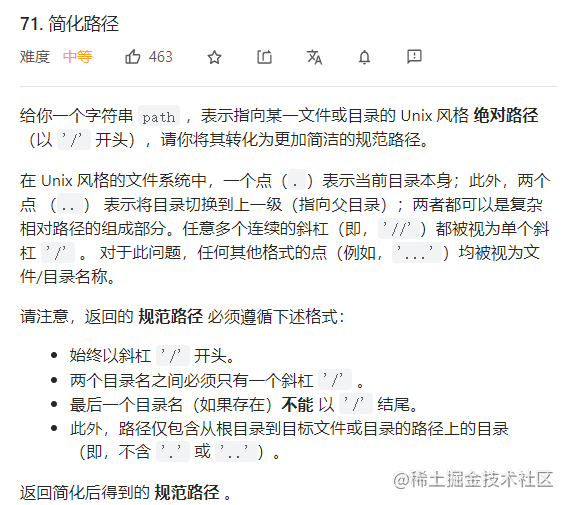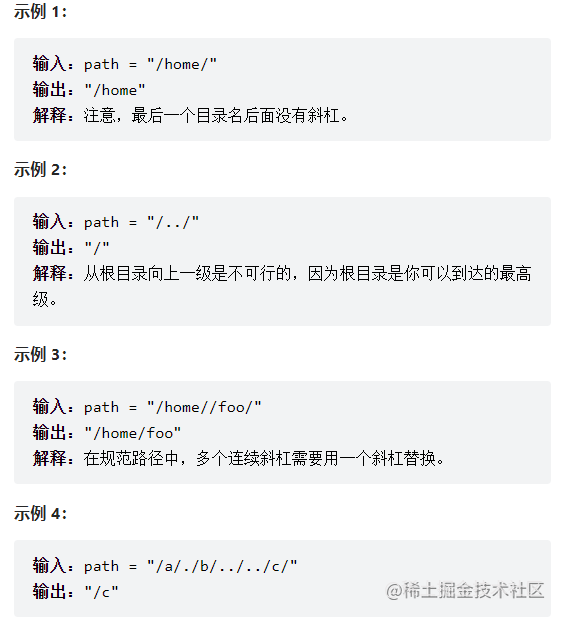## 解题思路​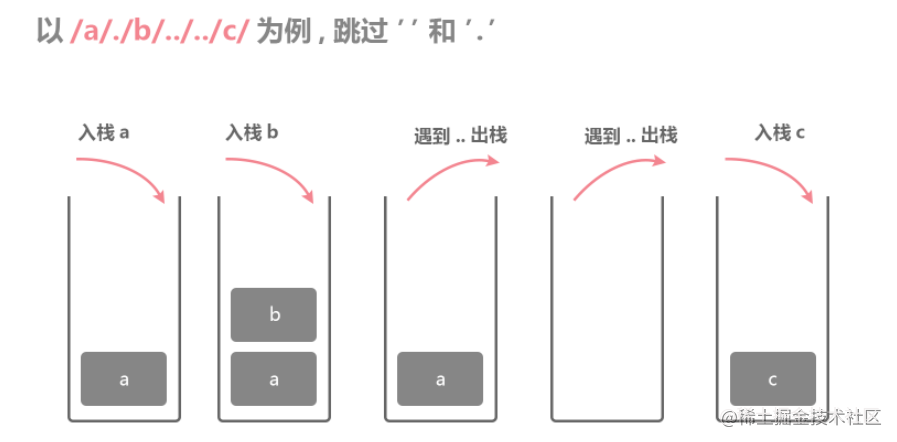## AC代码​

``var simplifyPath = function(path) {    // 简化路径的核心就是借助辅助栈    const stack = [];    // 分割字符串    const strArr = path.split('/');    // 遇到空字符串和一个点的直接跳过    // 遇到两个点则出栈    const res = [];    for (let v of strArr) {        if (v.length === 0 || v === '.') {            continue;        }        if (v === '..') {            stack.pop();            continue;        }        stack.push(v);    }    for (let i = 0; i < stack.length; i++) {        res.push('/');        res.push(stack[i]);    }    return res.length > 0 ? res.join('') : '/'};``

## 总结​

JustinLeetCode阅读需 1 分钟

## 题目描述​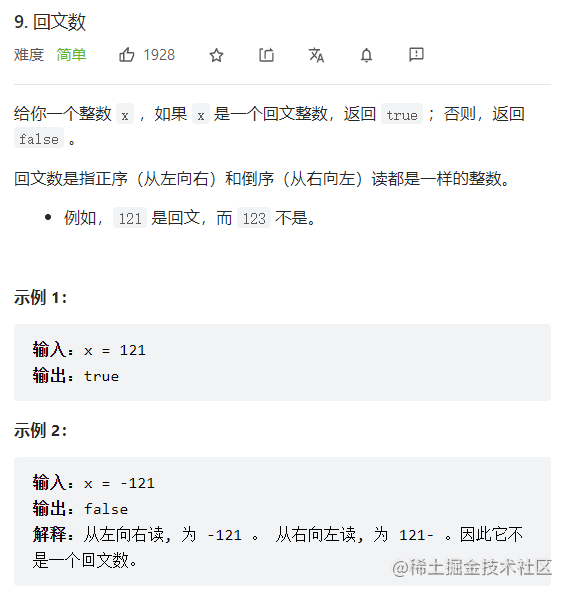## 解题思路​

### 思路一：反转比较法​

``var isPalindrome = function(x) {  // 使用反转对比的方法来判断是否是回文数字  x = x.toString();  const temp = x.split('').reverse();  const xArr = x.split('');  for (let i = 0; i < xArr.length; i++) {    if (temp[i] != xArr[i]) {      return false;    }  }  return true;};``

### 思路二：使用递归比较首尾元素​

``var isPalindrome = function(x) {  // 使用递归判断  // 递归的介绍条件是输入的x小于等于1  if (x.toString().length <= 1) {    return true;  }  x = x.toString().split('');  let start = 0;  let end = x.length - 1;  if (x[start] === x[end]) {    return isPalindrome(x.slice(1,end).join(''))  } else {    return false;  }};``

## 总结​

JustinLeetCode阅读需 1 分钟

## 题目描述​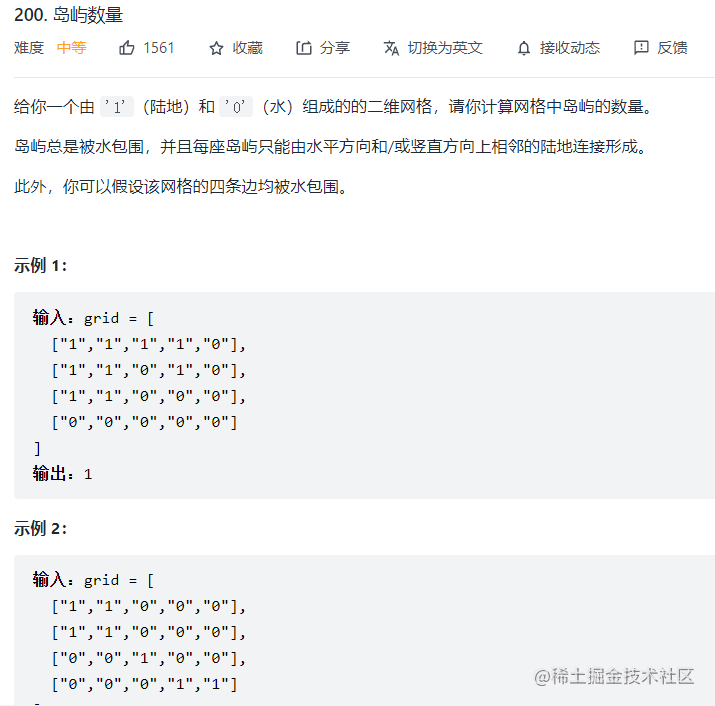## 解题思路​

### RQ1：怎么判断是一个岛屿？​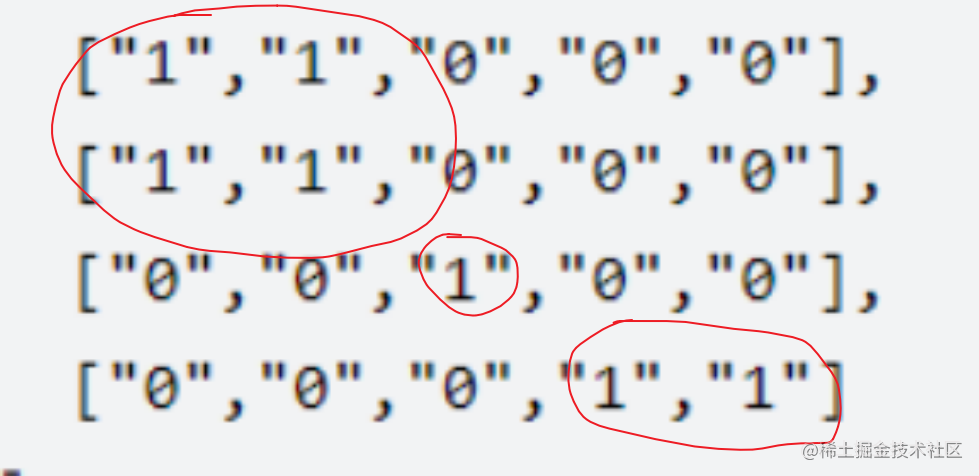## 解题代码​

``var numIslands = function (grid) {    // 岛屿的数量是一道经典的DFS问题    let row = grid.length;    let column = grid.length;    let count = 0;    for (let i = 0; i < row; i++) {        for (let j = 0; j < column; j++) {            if (grid[i][j] === '1') {                count++;                dfs(i,j,grid);            }        }    }    return count;    function dfs(i,j,grid) {        if (i < 0 || i >= row || j < 0 || j >= column || grid[i][j] === '0') {            return;        }        grid[i][j] = '0';        dfs(i+1,j,grid);        dfs(i,j+1,grid);        dfs(i-1,j,grid);        dfs(i,j-1,grid);    }};``

## 同类对比​

JustinLeetCode阅读需 2 分钟

## 题目描述​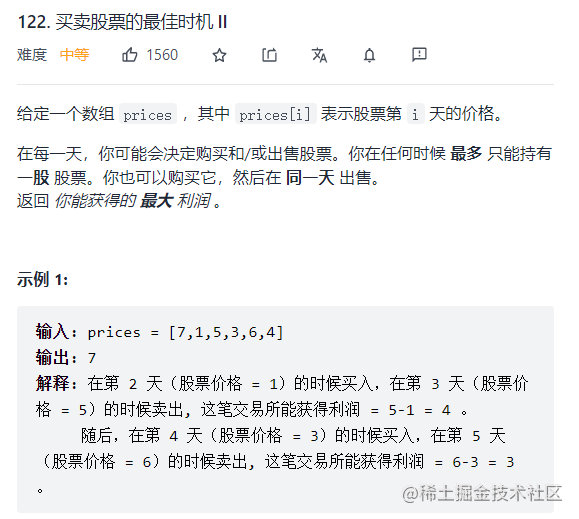## 解题思路​

• dp[i]：表示的是第i天，手上没有股票获取的最大收益，也就是说赚的钱数。
• dp[i]：表示的是第i天，手上有股票获取的最大收益。
• dp[i]的可能性：
• 第i-1天手里也没有股票：dp[i-1]
• 第i-1天手里有股票，但是今天卖了：dp[i-1] + prices[i]
• dp[i]的可能性：
• 前一天也有股票，并保持到今天：dp[i-1]
• 前一天没有股票，今天买入了：dp[i-1] - prices[i]

`手上没有股票的收益大，因为最后一天持有股票说明还没有变现。`

## AC代码实现​

``var maxProfit = function(prices) {  // 动态规划是解决买卖股票的最佳时机的核心技巧  // 首先构造一个二维数组dp  const dp = new Array(prices.length).fill([0,0]);  // 初始化  dp = 0;  dp = -prices;  // 处理一般情况  for (let i = 1; i < dp.length; i++) {    dp[i] = Math.max(dp[i-1],dp[i-1] + prices[i]);    dp[i] = Math.max(dp[i-1] - prices[i],dp[i-1]);  }  return dp[prices.length-1];};``

## 买卖股票的最佳时机I和买卖股票的最佳时机II的区别在哪里？​

``dp[i] = Math.max(- prices[i], dp[i - 1]);``

``dp[i] = Math.max(dp[i-1] - prices[i],dp[i-1]);``

## 总结​

JustinLeetCode阅读需 3 分钟

## 题目描述​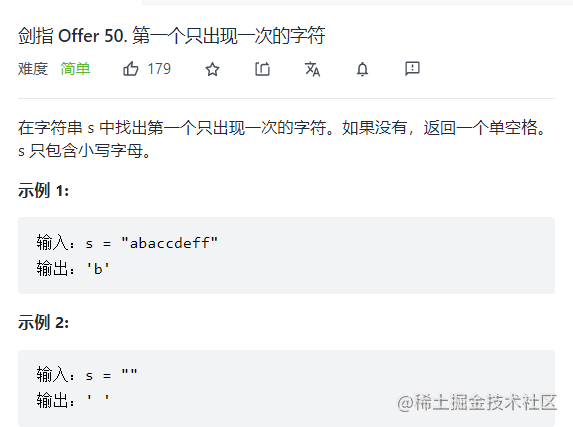## 解题思路​

1. 如果传入的参数是一个空字符串，则染回单空格。
2. 初始化一个map，遍历每一个字符，并更新字符出现的次数。
3. 遍历map的每一个item，如果item等于1，则直接返回item
4. 如果第三步没有找到符合条件的则返回单空格。

## AC代码​

``var firstUniqChar = function (s) {  if (s.length === 0) return " "  const map = new Map();  for (let v of s) {    if (map.has(v)) {      map.set(v,map.get(v) + 1);    } else {      map.set(v,1);    }  }  for (let item of map) {    if (item === 1) {      return item;    }  }  return " "};``

## 总结​

JustinLeetCode阅读需 2 分钟

## 题目描述​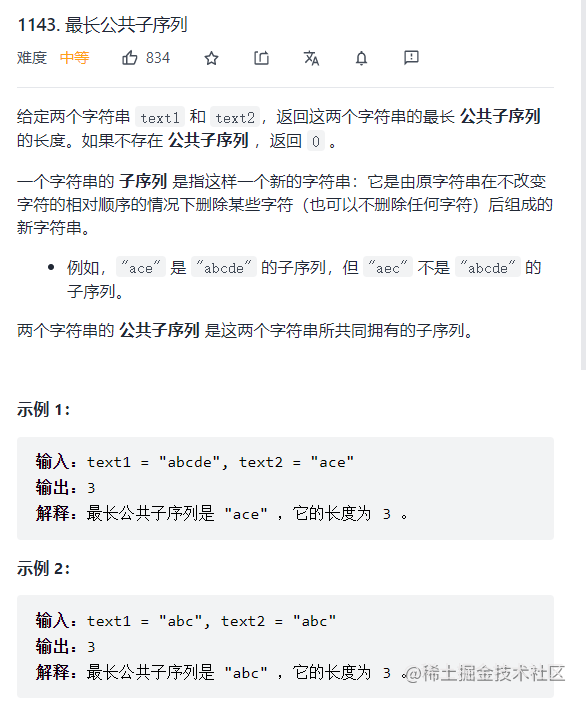## 解题思路​

• 要比较的字符相等
• dp[i][j] = dp[i-1][j-1] + 1
• 要比较的字符不相等
• dp[i][j] = Math.max(dp[i-1][j],dp[i][j-1])

## AC代码​

``var longestCommonSubsequence = function(text1, text2) {  // 使用表格法解决最长公共子序列  let row,column;  if (text1.length > text2.length) {    row = text2.length + 1;    column = text1.length + 1;  } else {    row = text1.length + 1;    column = text2.length + 1;    let temp = text1;    text1 = text2;    text2 = temp;  }  // 首先进行填零操作  const dp = [];  for (let i = 0; i < row; i++) {    dp[i] = new Array(column).fill(0)  }  // 从第一行的第一列开始进行遍历  for (let i = 1; i < row; i++) {    for (let j = 1; j < column; j++) {      if (text1[j-1] === text2[i-1]) {        dp[i][j] = dp[i-1][j-1] + 1;      } else {        dp[i][j] = Math.max(dp[i-1][j],dp[i][j-1]);      }    }  }  return dp[row-1][column-1];};``

1. 哪个字符串的长度长，哪个字符串的长度+1做列。
2. 如果是text2比较长，text1和text2需要进行交换。
3. 构造二维数组的时候fill中填入的一定要是一个常量，否则可能会出错。

## 启示​

JustinLeetCode阅读需 2 分钟

## 题目描述​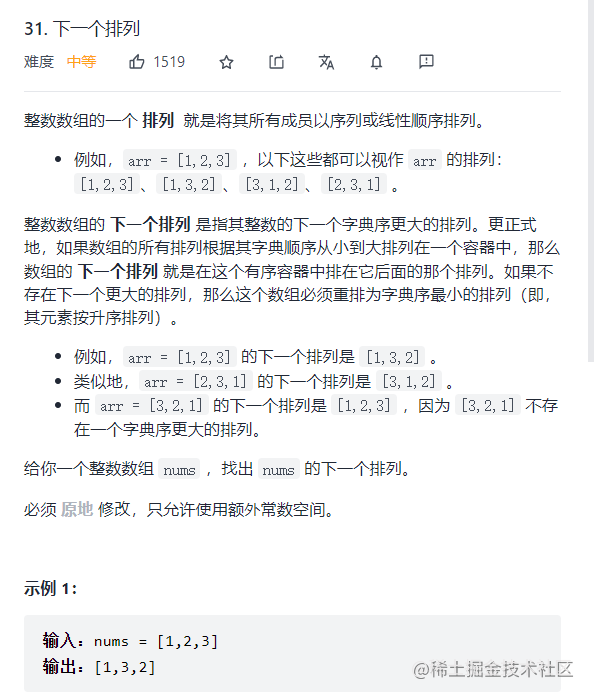## AC代码​

``var nextPermutation = function(nums) {  // 下一个排列要求的是原地排序  let flag = false;  let right = nums.length - 1;  // 第一步：从右往左进行遍历，查找是否有降序元素的存在  while (right) {    if (nums[right] > nums[right-1]) {      flag = true;      right--;      break;    } else {      right--;    }  }  if (flag) {    const sorted = nums.splice(right + 1).sort((next,pre) => next - pre);    // 找到排序好的数组的第一个比nums[right]的元素，然后进行交换    let temp;    for (let i = 0; i < sorted.length; i++) {      if (sorted[i] > nums[right]) {        temp = i;        break;      }    }    let t = nums[right];    nums[right] = sorted[temp];    sorted[temp] = t;    // 对sorted进行二次排序    sorted.sort((next,pre) => next - pre);    // 进行拼接    nums.push(...sorted);  } else {    nums.sort((next,pre) => next - pre)  }};``

## 参考文献​

JustinLeetCode阅读需 2 分钟

## 题目描述​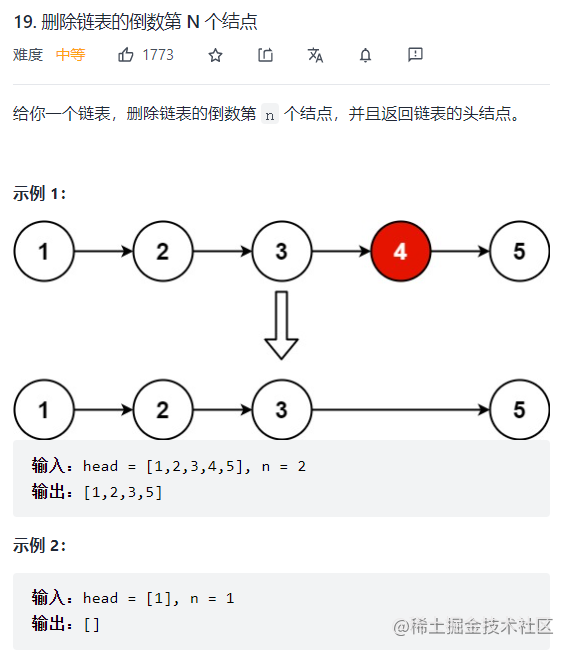## 解题思路​

### 快慢指针为什么能够找到链表的倒数第N个节点​

• 快指针走到了null，此时如果还没有走完n + 1个节点
• 让满秩阵继续走完剩余的节点数量，然后返回慢指针即可。
• 快指针走到了倒数第N个节点
• 此时快慢指针同时继续走，一旦快指针走到了null，删除慢指针的下一个节点，然后返回头指针即可。

## 解题代码​

``var removeNthFromEnd = function(head, n) {  // 删除链表的倒数第N个节点是一个典型的双指针问题  let slow = head;  let fast = head;  let temp = head;  // 第一步：让快指针先走n步  let count = n+1;  while (count && fast) {    fast = fast.next;    count--;  }  if (!fast && count) {    while (count) {      slow = slow.next;      count--;    }    return slow;  }  while (fast) {    fast = fast.next;    slow = slow.next;  }  let stemp = slow.next.next;  slow.next = stemp;  return temp;};``

## 题目反思​

JustinLeetCode阅读需 2 分钟

## 题目描述​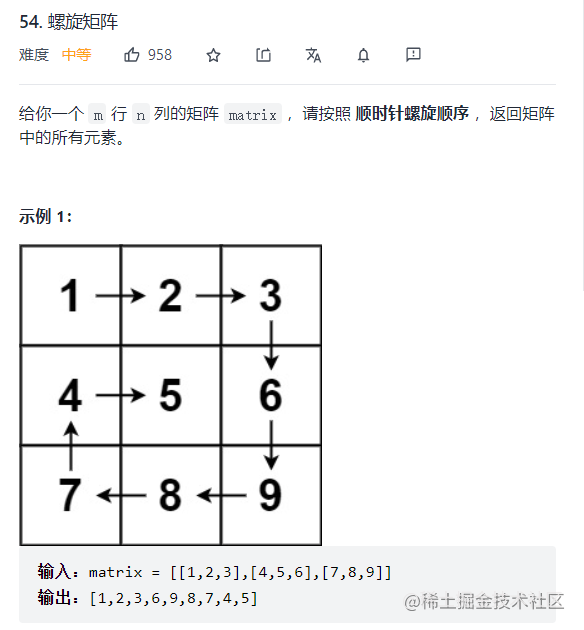## 解题思路​

1. 首先定义四个指针，指向如下图所示：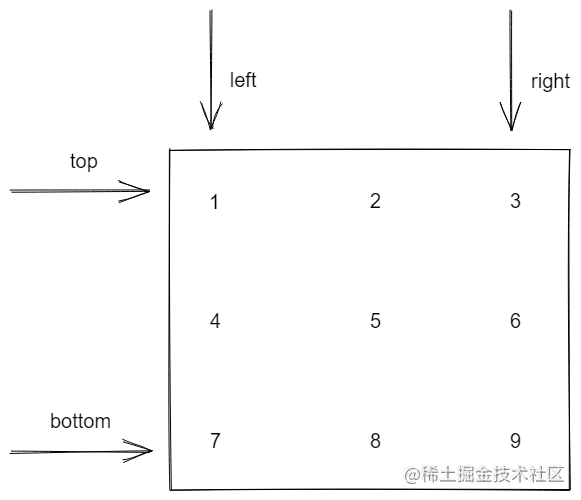1. 按照顺时针进行遍历，分别是从左到右、从上到下、从右到左、从下到上的思路。

2. 一轮循环后让左指针+1，继续下一轮循环，需要注意的是每次移动指针的时候，都需要注意指针是否越界。

``var spiralOrder = function(matrix) {  // 螺旋矩阵的核心是使用四个指针来辅助遍历  // 首先是初始条件的判断，如果矩阵的长度为0，则返回空数组  if (matrix.length === 0) return [];  // 定义四个指针  let left = 0;  let top = 0;  let right = matrix.length - 1;  let bottom = matrix.length - 1;  // 定义最终返回的结果  const res = [];  // 开始遍历  while (1) {    // 主要是按照顺时针进行遍历    // 第一步：从左到右    for (let i = left; i <= right; i++) {      res.push(matrix[top][i])    }    // 第二步：从上到下    top++;    if (top > bottom) break;    for (let i = top; i <= bottom; i++) {      res.push(matrix[i][right]);    }    // 第三步：从右到左    right--;    if (right < left) break;    for (let i = right; i >= left; i--) {      res.push(matrix[bottom][i])    };    // 第四步：从下到上    bottom--;    if (bottom < top) break;    for (let i = bottom; i >= top; i--) {      res.push(matrix[i][left])    }    // 第五步：也是最容易被遗忘的一步    left++;    if (left > right) break;  }  return res;};``

## 题目反思​

JustinLeetCode阅读需 2 分钟

## 题目描述​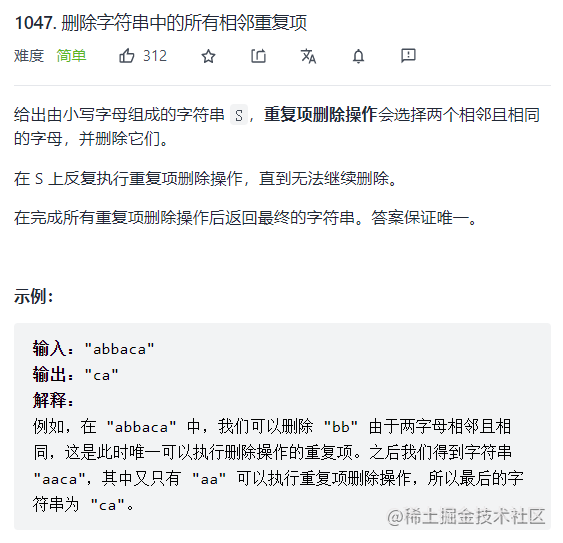## 解题思路​

``var removeDuplicates = function(s) {  // 这道题目的思想类似于打牌的思路，区别在于本题是通过栈来实现打牌思路  // 有机会可以将这道题目和最长回文串这道题目进行归纳总结  const stack = [];  for (let i = 0; i < s.length; i++) {    if (stack.length && stack[stack.length - 1] === s[i]) {      stack.pop();    } else {      stack.push(s[i]);    }  }  return stack.join('');};``

## 题目反思​

JustinLeetCode阅读需 2 分钟